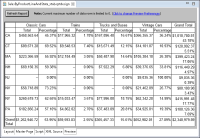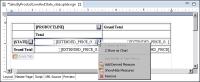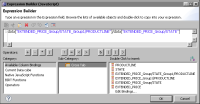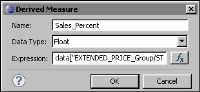Typically, measures are field values that are aggregated using one of the standard aggregate functions. You can also define a measure, called a derived measure, that involves a more complex calculation. For example, instead of displaying just sales totals by product line and by territory, the cross tab can also display the product line sales totals as a percentage of the territory total, as shown in Figure 24‑45.Figure 24‑45 Cross tab displaying two measures, one a derived measure calculating product line totals as a percent of the state total
How to create a derived measure
1 Click the button next to a data element that displays a measure, as shown in Figure 24‑46.Figure 24‑46 Displaying a menu for a selected measure in the cross tabIn Name, type a name for the derived measure.In Data Type, select a data type appropriate for the data returned by the expression you specify next. For a derived measure, you typically select Float or Integer.In Expression, specify the expression that evaluates to the data to return. Use the expression builder to construct the expression. Figure 24‑47 shows an example of an expression for calculating the product line sales total as a percentage of the state total. The expression uses column bindings defined in the cross tab.